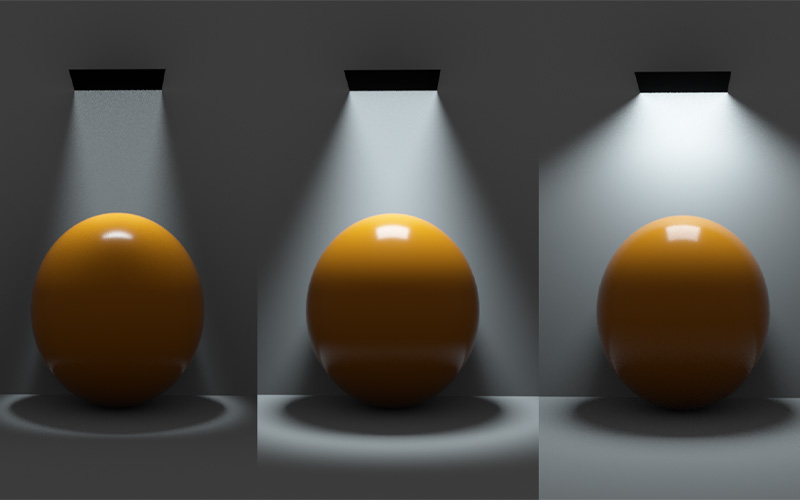## OSL | 灯光扩散

446 0 2021-05-10

OSL连接给灯光的Distribution通道

``````shader Spotlight(
float hardDeg = 40 [[ string label = "Hard angle", float min = 1, float max = 360, float sensitivity = .1]],
float softDeg = 40 [[ string label = "Soft angle", float min = 0, float max = 360, float sensitivity = .1]],
int type = 2 [[string widget="mapper", string label = "Direction mode",
string options = "Target:1|Away from target:2|Direction:3|Direction (object space):4|Normal space:5"]],
point direction = point(0, -1, 0) [[string label = "Direction / Target"]],
float minBrightness = 0 [[ string label = "Brightness outside cone", float min = 0, float max = 1, float sliderexponent=4]],
int compensate = 0 [[ string label = "Keep lobe power", string widget = "boolean"]],
output color c = 0)
{
vector ref = (type == 5) ? N : direction;
if (type == 1)
{
ref = ref - P;
}
if (type == 2)
{
ref = P - ref;
}
if (ref == vector(0, 0, 0)) {
// 0, 0, 0 -> provide sensible defaults for directions
if (type == 3 || type == 4) ref = vector(0, -1, 0);
}
if (type == 4)
{
ref = transform("object", "world", ref);
}
vector dir = normalize(I);
ref = normalize(ref);
float hardLimit = radians(hardDeg) * .5;
float softLimit = radians(softDeg) * .5;
float angle = acos(dot(-dir, ref));
c = 1 - smoothstep(hardLimit, hardLimit + softLimit, angle);
// the cosine lobe integral is π, but a smaller lobe will have
// less total power. Scale our value up so total power remains
// approximately constant
if (compensate) {
float appxHeight = 1 - cos(hardLimit + softLimit);
if (appxHeight < 1) c /= appxHeight;
}
c = minBrightness + (1 - minBrightness) * c;
}``````